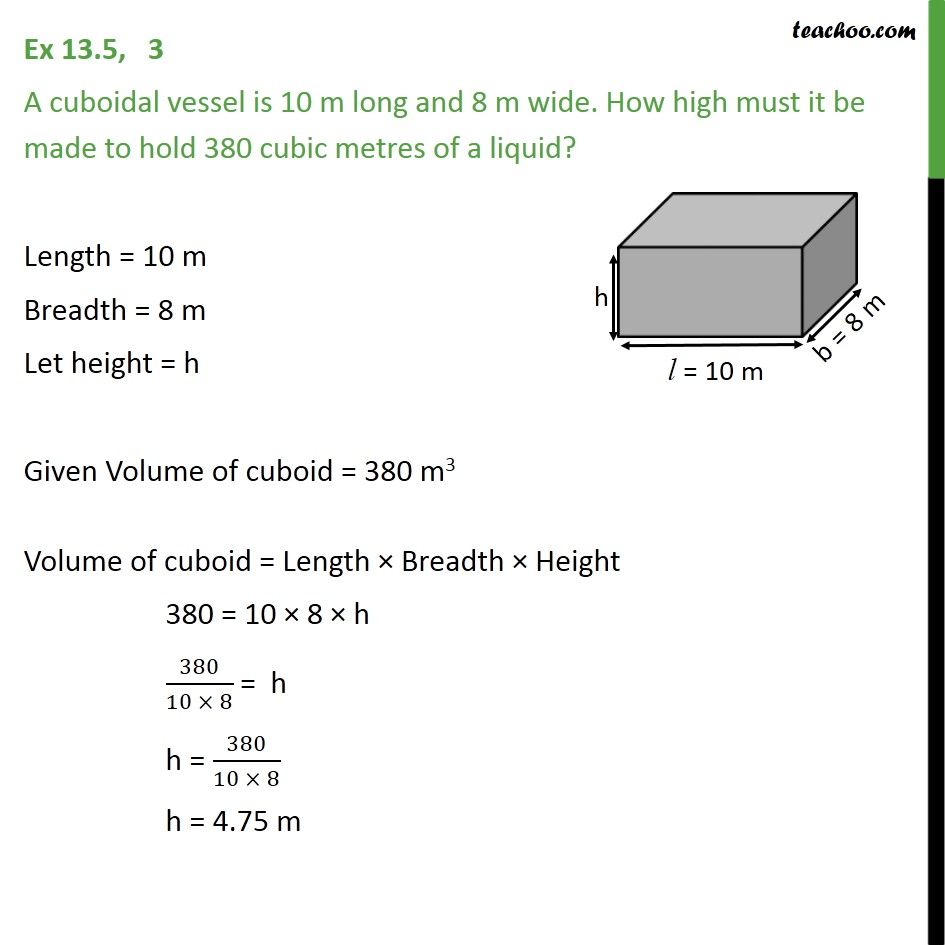Volume of Cube and Cuboid

Chapter 11 Class 9 Surface Areas and Volumes
Serial order wiseLearn in your speed, with individual attention - Teachoo Maths 1-on-1 Class

### Transcript

Question 3 A cuboidal vessel is 10 m long and 8 m wide. How high must it be made to hold 380 cubic metres of a liquid? Length = 10 m Breadth = 8 m Let height = h Given Volume of cuboid = 380 m3 Volume of cuboid = Length × Breadth × Height 380 = 10 × 8 × h 380/(10 × 8) = h h = 380/(10 × 8) h = 4.75 m